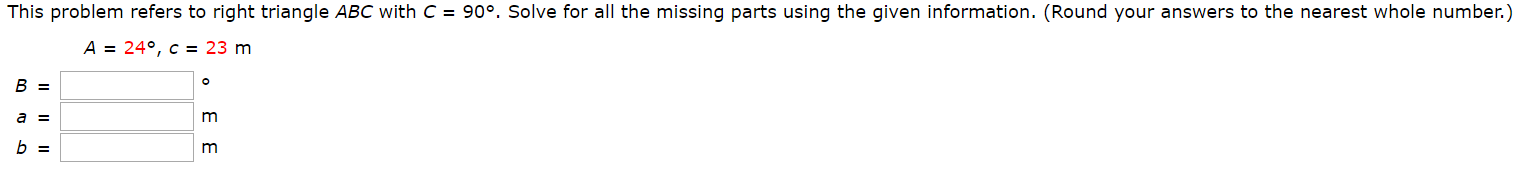# This problem refers to right triangle ABC with C90°. Solve for all the missing parts using the given information. (Round your answers to the nearest whole number.)A = 24°, c = 23 mB =a =m3 3 This problem refers to right triangle ABC with C90°. Solve for all the missing parts using the given information. (Round your answers to the nearest whole number.)A = 24°, c = 23 mB =a =m3 3

Question

Photo attachedhelp_outlineImage TranscriptioncloseThis problem refers to right triangle ABC with C 90°. Solve for all the missing parts using the given information. (Round your answers to the nearest whole number.) A = 24°, c = 23 m B = a = m 3 3 fullscreenhelp_outlineImage TranscriptioncloseThis problem refers to right triangle ABC with C 90°. Solve for all the missing parts using the given information. (Round your answers to the nearest whole number.) A = 24°, c = 23 m B = a = m 3 3 fullscreen
check_circleExpert Solution
Step 1

Given,

Step 2

The diagrammatic representation is,

Step 3

Therefore, in triangle ...

### Want to see the full answer?

See Solution

#### Want to see this answer and more?

Solutions are written by subject experts who are available 24/7. Questions are typically answered within 1 hour*

See Solution
*Response times may vary by subject and question
Tagged in

### Trigonometric Ratios